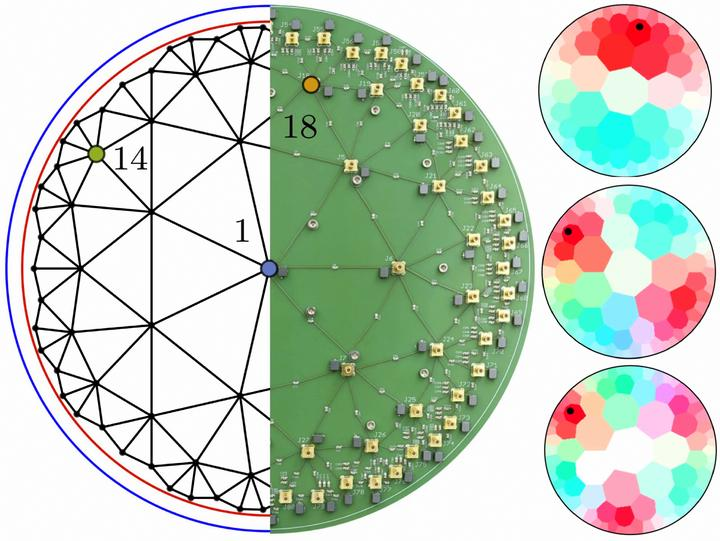# Simulating hyperbolic space on a circuit board### Abstract

The Laplace operator encodes the behavior of physical systems at vastly different scales, describing heat flow, fluids, as well as electric, gravitational, and quantum fields. A key input for the Laplace equation is the curvature of space. Here we discuss and experimentally demonstrate that the spectral ordering of Laplacian eigenstates for hyperbolic (negatively curved) and flat two-dimensional spaces has a universally different structure. We use a lattice regularization of hyperbolic space in an electric-circuit network to measure the eigenstates of a ‘hyperbolic drum’, and in a time-resolved experiment we verify signal propagation along the curved geodesics. Our experiments showcase both a versatile platform to emulate hyperbolic lattices in tabletop experiments, and a set of methods to verify the effective hyperbolic metric in this and other platforms. The presented techniques can be utilized to explore novel aspects of both classical and quantum dynamics in negatively curved spaces, and to realise the emerging models of topological hyperbolic matter.

Publication
Nat. Commun. 13, 4373 (2022)